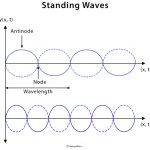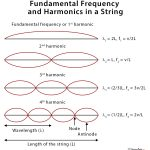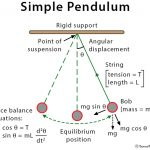# Prism

## Definition: What is a Prism?

A prism is a transparent 3-dimensional object made of glass and surrounded by intersecting planes, some of which are identical to one another. The most critical parameters of a prism are the angle and material that determines its purpose. While prism has a long history, the best-known experiment using prism was performed by Issac Newton in 1666, when he studied the dispersion of light.

## What Does a Prism Do?

A prism is used to analyze, bend, and disperse light resulting in the appearance of a rainbow.

## How Does a Prism Work?

Each color, or wavelength, making up the white light is bent or refracted to a different amount. The shorter wavelengths (violet end of the spectrum) bend the most, and the longer wavelengths (red end of the spectrum) bend the least. Thus, a pattern of different colors appears on a screen.

## How to Use a Prism?

The best way to use a prism is to keep it in front of a light source, like sunlight and incandescent white light bulb and a screen behind it. The prism will disperse white light into its constituent colors, which gives the appearance of a rainbow when observed on the screen.

## Shapes of Prism

The shapes of the bases usually classify prisms. Some common shapes of the base are triangular, square, rectangular, hexagonal, and parallelogram.

### Rectangular prism

A rectangular prism is a solid 3-dimensional object which has six faces (sides) that are rectangles. Aside, it has 12 edges and eight vertices.  It is also called a cuboid. The surface area of a rectangular prism is the product of its length and breadth, and its volume is the product of its length, breadth, and height.

### Triangular Prism

A triangular prism is a polyhedron made up of two triangular bases and three sides, making that five faces. Further, it has six vertices and nine edges. The two triangular bases are parallel and congruent to each other, whose area is one-half times the product of the base length and altitude. The surface area of a triangular prism is the sum of the areas of the sides and bases. The volume is the product of the area of the base and the thickness of the prism.

Triangular prisms made to disperse light are usually made with bases that are equilateral triangles so that the angles between adjacent sides of the prism are 60°. This type of prism is called refracting prism. Some prisms have bases that are isosceles right triangles (45° – 90° – 45°) and reflect light using the principle of total internal reflection.

Image Courtesy: Fineartamerica.com

### Hexagonal Prism

A hexagonal prism is composed of two hexagonal (six-sided) bases and six rectangular sides, making it a polyhedron with eight faces, 18 edges, and 12 vertices. The surface area of a hexagonal prism is the sum of the areas of all the sides and bases. The volume is the product of the basal area and the thickness of the prism.

### Trapezoidal Prism

A trapezoidal prism consists of two congruent trapezoids that are connected by four rectangles. The two congruent trapezoids are its top and lower base. Trapezoids are quadrilaterals (four-sided figures) that have only one pair of parallel lines. The four rectangles that connect the corresponding sides of the two bases are called the lateral faces of the prism. The surface area of a trapezoidal prism is the sum of the areas of all the sides and bases. The volume is the product of the basal area and the thickness of the prism.

## Applications of Prism

• In splitting, polarization, depolarization, and image orientation in refractometer and spectrographic instruments
• In certain spectroscopes (instruments for analyzing light) for determining the identity and structure of materials that emit or absorb light
• Eye care professionals use prism correction as a component of some eyeglass prescriptions for the treatment of strabismus

## FAQs

Q1. How to find the surface area of a prism?

Ans. The surface area of a prism can be found by summing the areas of all its sides and base.

Q2. How to find the volume of a prism?

Ans. The volume of a prism can be found by taking the area of its base and multiplying it with the prism’s thickness.

Q3. How to find the perimeter of a prism?

Ans. The perimeter of a prism can be found by summing the length of all its edges.

Q4. Is cube a prism?

Ans. Yes. A cube is a particular case of a rectangular prism.

Q5. Is a cylinder a prism?

Ans. A cylinder is not a prism, because it has curved sides.

Q6. How many edges does a pentagonal prism have?

Ans. A pentagonal prism has 15 edges.

Q7. How many faces does a pentagonal prism have?

Ans. A pentagonal prism has seven faces.

Q8. Is a cone a pyramid or prism?

Ans. A cone is a pyramid. It cannot be a prism as it has curved sides.

Article was last reviewed on Wednesday, June 3, 2020

### Related articlesStanding WavesFundamental FrequencySimple PendulumKirchhoff’s Law